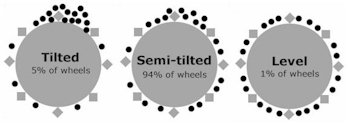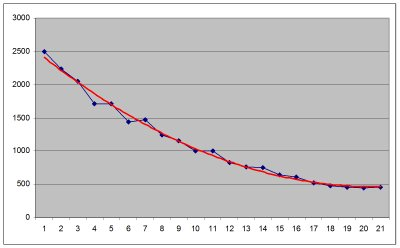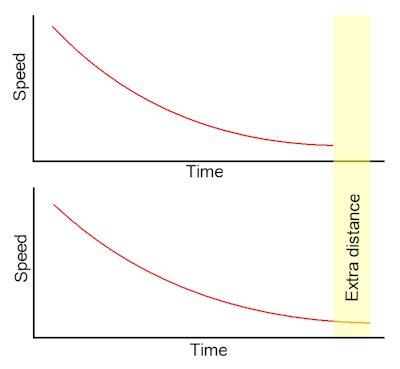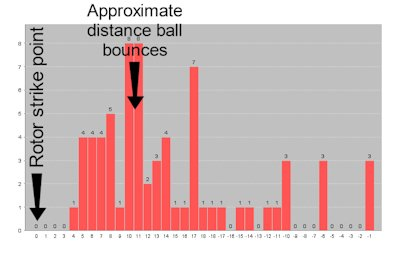### Why Some Computers Are Ineffective

Generally you get what you pay for. There are some major differences between computers that affect overall accuracy and practicality. Below are some of the problems roulette computers must overcome:

Problem 1: HOW the ball will fall

Especially on modern wheels, the ball does not hit a vertical diamond, then be deflected predictably down on nearly every spin. This may have been the case with wheels 10 years ago, but it isn't anymore. On modern wheels, even if a computer correctly predicts where the ball will lose momentum and fall, predictions will not be accurate. Why not? Because the ball may hit a horizontal diamond and be deflected to the other side of the wheel. The ball may clip a horizontal diamond, then hit a vertical diamond. The ball may hit a vertical diamond solidly. It may even completely miss all diamonds. Whatever the case may be, the different types of ball falls often have completely different outcomes.

Putting this into perspective, for a computer to beat modern wheels, it must know not only where the ball will fall, but also HOW it will fall. Our computers are only ones available that determine not only which diamond will be hit, but which part of the diamond will be hit, and the resulting manner in which the ball falls.

Problem 2: WHERE the ball will fall

Ball tracks have many imperfections that appear subtle, but they actually have an enormous influence on where the ball will actually fall from the ball track. Every roulette computer except ours assumes the ball will either:

 a. Fall from the ball track at one particular point most times (called a "tilted" wheel and they are quite rare), OR b. That the wheel is absolutely perfect and that the ball will simply fall from the ball track merely when it reaches a certain speed.

There are numerous factors that determine where the ball is going to fall, and when. See below the three types of wheels you will encounter:LEFT: The black dots represent where the ball loses momentum and falls from the ball track for each type of wheel (this is just where the ball loses momentum and falls, NOT where it actually strikes the rotor). Heavily tilted wheels are very difficult to find, and level wheels are even rarer. Almost every wheel is semi-tilted, and on such wheels, every computer except ours has a dramatically reduced edge. Specifically that is because 94% of wheels are too tilted to be considered level, but too level to be considered tilted. In most cases, the resulting accuracy for simplistic computers is no better than random.

Problem 3: Air pressure and ball deceleration rate variations

In any environment, air pressure gradually varies over time. When air pressure varies, the deceleration rate of the ball, AND the speed at which the fall falls from the ball track also varies. This means the overall distance the ball will roll will also vary. A player can no more change air pressure than they can change the weather. Significant air pressure variations often occur within approximately 30 minutes, but the change occurs gradually.

Consider the following example: if the ball was released at a particular speed, the ball may complete 18 revolutions before falling. Now if the ball is released at the exact same speed just one hour later, even a very slight air pressure variation may mean the ball will complete 21.3 revolutions before falling - that's a difference of 3.3 whole revolutions, and about 4 extra seconds before the ball falls.

Here's how this will affect affect accuracy if the computer does NOT account for the air pressure variations:

 i. If the wheel is tilted: the computer will have an error of about 4 seconds. But the degree of error will not remain consistent, so accuracy rates will gradually decline. When the computer attempts to determine what pocket will be under the dominant ball drop zone when the ball falls, the computer can be way off target. On most tilted wheels, you will still have an edge, but the edge may be half of what is possible. ii. If the wheel is level: results would be disastrous - the computer will be up to 3.3 whole revolutions off-target. If the degree of error was consistent, there wouldn't be a problem. But in reality the degree of error will change: from 0.1, to 0.2, to 0.3, etc. In such a case, achieving any kind of accuracy would be virtually impossible. This is not even taking into account the various other points raised on this page, in particularly than a discrepancy of even 1cm may make it impossible for the computer to know which diamond will be hit, and which part of the diamond will be hit. iii. If the wheel is semi-tilted: basically much the same as the level wheel, the degree of error would vary. The results would likely be no better than random.

For a computer to make adjustments for air pressure variation, it must use polynomials. Polynomials are mathematical equations used to generate a "best fit" curve to model the deceleration of the ball.LEFT: The blue dots represent the actual ball timings. the red line is the "best fit" polynomial curve. The polynomial curve can be seen on our computers in the settings. You can see that despite the obvious timing errors (blue dots) the polynomial curve is smooth and accurate.

As air pressure naturally varies in the casino environment, the ball's deceleration rate will naturally change too. Even a very slight change in ball deceleration rate may make the ball travel several additional revolutions. See the chart below for explanation:LEFT: In both of these charts, the ball is released at exactly the same speed. The difference in air pressure and therefore the ball deceleration rate is only marginal between each chart. However, even very small deceleration rates make a large difference to the overall distance traveled by the ball.

The yellow area shows the additional difference traveled. Within only 30 spins in the casino (about an hour), this difference in distance is often over 3 revolutions.

Problem 4: Varying ball bounce and scatter with different wheel speeds

When the wheel spins at different speeds, the ball of course behaves differently. In fact, even an imperceivable difference in wheel speed may mean the ball bounces to the opposite side of the predicted area on the wheel.

For now let'sassume a computer perfectly predicts where the ball falls, and how it falls. So let's assume the computer knows exactly which pocket the ball will first hit when it falls. Of course the ball will bounce off the initial pocket before coming to rest elsewhere, but for now we're just talking about where the ball first hits on the rotor (called the "rotor strike point").

With the exception of our computer, every computer assumes the ball will bounce the same distance from the rotor strike point irrespective of the wheel rotor speed. So let's say we charted the distance between the rotor strike point to where the ball actually landed, we would expect the chart to look like below:LEFT: This is called a ball scatter chart. On the horizontal axis, the value of "0" is where the ball actually hits the wheel rotor (the part with the pockets). You can see the ball bounces and comes to rest approximately 10 pockets from the rotor strike point. Most roulette computers conduct analysis based on the assumption that the wheel rotor speed is exactly the same every time. This incorrect assumption alone often makes some roulette computers completely ineffective.

So while a typical scatter chart initially sounds good in theory, the reality is when the wheel is at different speeds, the ball may behave completely differently. Especially on modern wheels, even the slightest difference in wheel speed can mean the ball bounces an extra 12 or more pockets - modern are designed this way. In such cases, if the computer assumes the wheel is spinning at the same speed every time, accuracy may be no better than random. Our most advanced computers automatically adjust predictions based on the wheel speed of individual spins.

You get what you pay for:

Our roulette computers are the only ones that correctly address all of the above problems. We do not develop our technology as a hobby, or with the primary purpose of selling it - we develop our technology primarily to use it ourselves, and it is a multi-million dollar business for us. We have invested considerable resources to ensure our technology is literally as good as it can be.

ShareThis Copy and Paste